挤压铝型材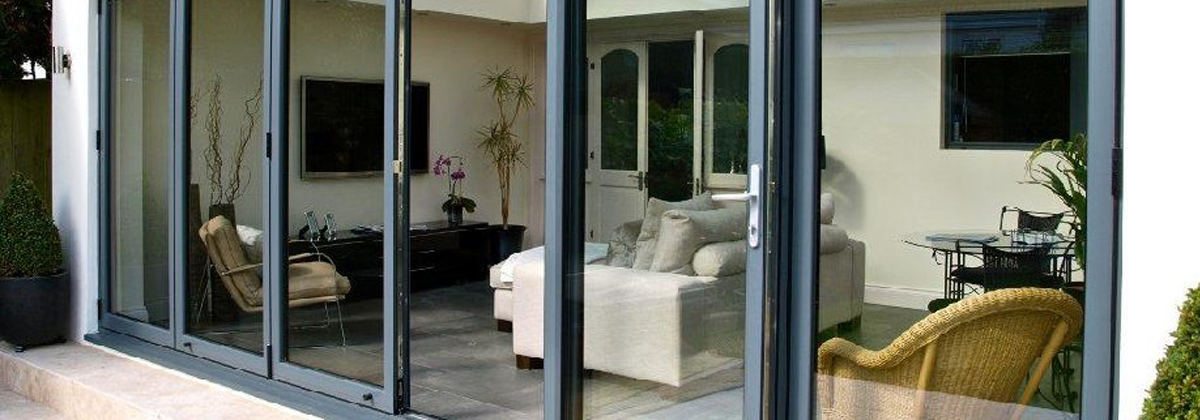+铝型材

• 百叶铝型材

铝百叶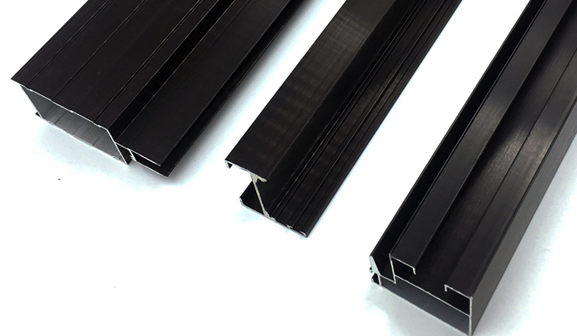• 原材料&热处理:铝合金& 6063-T5
• 表面处理:
• 厚度:0.4毫米及以上，或客户订制
• 颜色:
• 生产周期:大货20-25天，外加10天制作模具
• 长度:5.8米， 或客户订制
• OEM&ODM:支持OEM生产，贴膜版设计费用300\$或以上。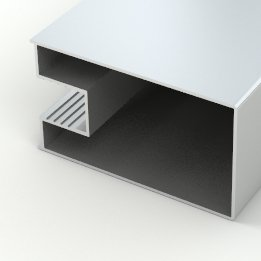FLB-001FLB-002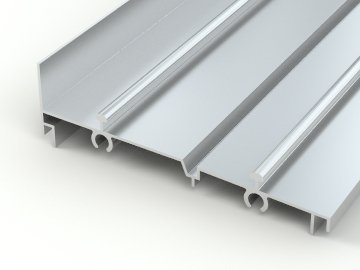FLB-003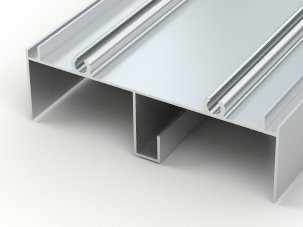FLB-004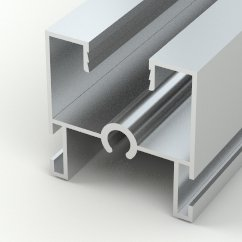FLB-005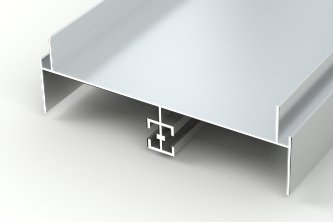FLB-006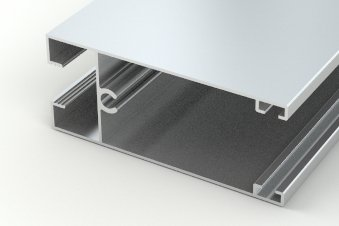FLB-007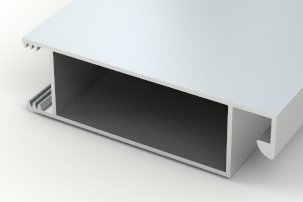FLB-008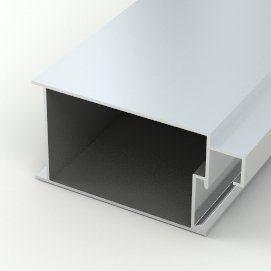FLB-033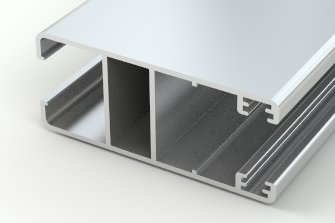FLB-060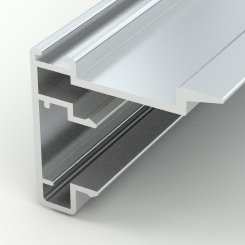FLB-061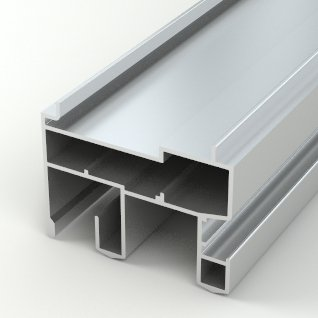FLB-062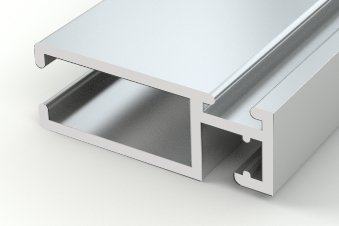FLB-063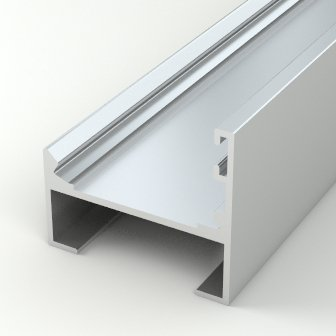FLB-065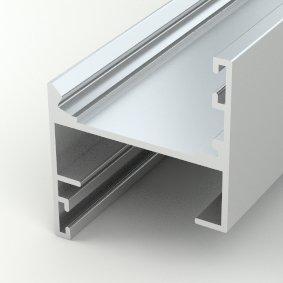FLB-066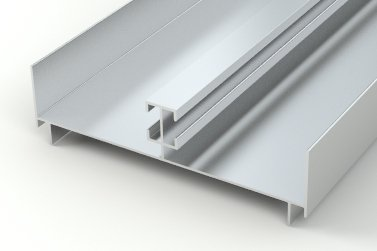FLB-069FLB-070FLB-071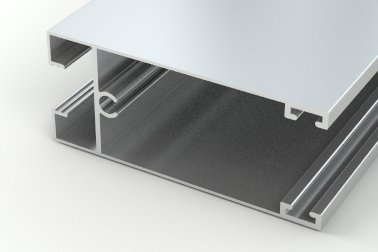FLB-074FLB-075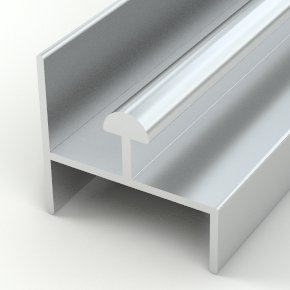FLB-076FLB-077FLB-078FLB-082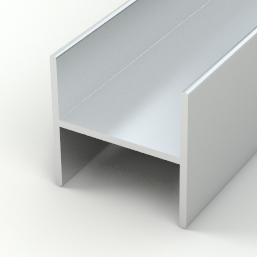FLB-083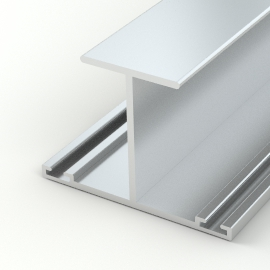FLB-085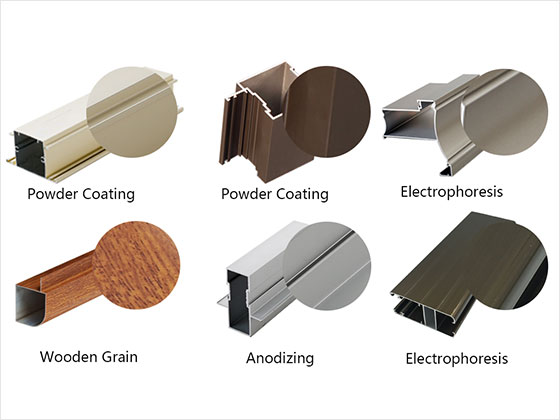提交

• ---------
• 阿富汗
• 阿尔巴尼亚
• 澳大利亚
• 巴西
• 加拿大
• 中国
• 法国
• 德国
• 意大利
• 西班牙
• 瑞士
• 英国
• 美国
• ---------
• 阿富汗
• 阿尔巴尼亚
• 阿尔及利亚
• 美属萨摩亚
• 安道​​尔
• 安哥拉
• 安圭拉
• 安提瓜和巴布达
• 阿根廷
• 亚美尼亚
• 阿鲁巴岛
• 澳大利亚
• 奥地利
• 阿塞拜疆
• 巴哈马
• 巴林
• 孟加拉国
• 巴巴多斯
• 白俄罗斯
• 比利时
• 伯利兹
• 贝宁
• 百慕大
• 不丹
• 玻利维亚
• 波斯尼亚和黑塞哥维那
• 博茨瓦纳
• 布维岛
• 巴西
• 英属印度洋领地
• 文莱达鲁萨兰国
• 保加利亚
• 布基纳法索
• 布隆迪
• 柬埔寨
• 喀麦隆
• 加拿大
• 佛得角
• 开曼群岛
• 中非共和国
• 乍得
• 智利
• 中国
• 圣诞岛
• 科科斯群岛
• 哥伦比亚
• 科摩罗
• 刚果
• 库克群岛
• 哥斯达黎加
• 科特迪瓦
• 古巴
• 塞浦路斯
• 捷克共和国
• 朝鲜人民民主共和国
• 刚果民主共和国
• 丹麦
• 吉布提
• 多明尼加
• 东帝汶
• 厄瓜多尔
• 埃及
• 萨尔瓦多
• 赤道几内亚
• 厄立特里亚
• 爱沙尼亚
• 埃塞俄比亚
• 福克兰群岛
• 法罗群岛
• 斐济
• 芬兰
• 法国
• 法属圭亚那
• 法属波利尼西亚
• 法国南部领土
• 加蓬
• 冈比亚
• 格鲁吉亚
• 德国
• 加纳
• 直布罗陀
• 希腊
• 格陵兰
• 格林纳达
• 瓜德罗普岛
• 关岛
• 危地马拉
• 几内亚
• 几内亚比绍
• 圭亚那
• 海地
• 赫德岛和麦克唐纳群岛
• 洪都拉斯
• 中国香港
• 匈牙利
• 冰岛
• 印度
• 印度尼西亚
• 伊朗
• 伊拉克
• 爱尔兰
• 以色列
• 意大利
• 牙买加
• 日本
• 约旦
• 哈萨克斯坦
• 肯尼亚
• 基里巴斯
• 韩国
• 科威特
• 吉尔吉斯共和国
• 老挝
• 拉脱维亚
• 黎巴嫩
• 莱索托
• 利比里亚
• 列支敦士登
• 立陶宛
• 卢森堡
• 中国澳门
• 马其顿
• 马达加斯加
• 马拉维
• 马来西亚
• 马尔代夫
• 马里
• 马耳他
• 马绍尔群岛
• 马提尼克
• 毛里塔尼亚
• 毛里求斯
• 马约特
• 墨西哥
• 密克罗尼西亚
• 摩尔多瓦
• 摩纳哥
• 蒙古
• 蒙特塞拉特
• 摩洛哥
• 莫桑比克
• 缅甸
• 纳米比亚
• 瑙鲁
• 尼泊尔
• 荷兰
• 新喀里多尼亚
• 新西兰
• 尼加拉瓜
• 尼日尔
• 尼日利亚
• 纽埃
• 诺福克岛
• 北马里亚纳群岛
• 挪威
• 阿曼
• 巴基斯坦
• 帕劳
• 巴勒斯坦
• 巴拿马
• 巴布亚新几内亚
• 巴拉圭
• 秘鲁
• 菲律宾
• 皮特凯恩群岛
• 波兰
• 葡萄牙
• 波多黎各
• 卡塔尔
• 留尼汪岛
• 罗马尼亚
• 俄国
• 卢旺达
• 圣赫勒拿
• 圣基茨和尼维斯
• 圣卢西亚
• 圣皮埃尔和密克隆
• 圣文森特和格林纳丁斯
• 圣马力诺
• 圣多美和普林西比
• 沙特阿拉伯
• 塞内加尔
• 塞尔维亚
• 塞舌尔
• 塞拉利昂
• 新加坡
• 斯洛伐克
• 斯洛文尼亚
• 越南社会主义共和国
• 所罗门群岛
• 索马里
• 南非
• 南乔治亚岛和南桑威奇群岛
• 西班牙
• 斯里兰卡
• 利比亚
• 苏丹
• 苏里南
• 斯瓦尔巴群岛和扬马延岛
• 斯威士兰
• 瑞典
• 瑞士
• 阿拉伯叙利亚共和国
• 中国台湾
• 塔吉克斯坦
• 坦桑尼亚
• 泰国
• 克罗地亚共和国
• 多哥共和国
• 托克劳
• 汤加
• 特立尼达和多巴哥
• 突尼斯
• 土耳其
• 土库曼斯坦
• 特克斯和凯科斯群岛
• 图瓦卢
• 乌干达
• 乌克兰
• 阿拉伯联合酋长国
• 英国
• 美国
• 美国本土外小岛屿
• 乌拉圭
• 美属维尔京群岛
• 乌兹别克斯坦
• 瓦努阿图
• 梵蒂冈城国
• 委内瑞拉
• 瓦利斯和富图纳群岛
• 西撒哈拉
• 萨摩亚
• 也门
• 赞比亚
• 津巴布韦

下载前请填写表格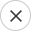• ---------
• 阿富汗
• 阿尔巴尼亚
• 澳大利亚
• 巴西
• 加拿大
• 中国
• 法国
• 德国
• 意大利
• 西班牙
• 瑞士
• 英国
• 美国
• ---------
• 阿富汗
• 阿尔巴尼亚
• 阿尔及利亚
• 美属萨摩亚
• 安道​​尔
• 安哥拉
• 安圭拉
• 安提瓜和巴布达
• 阿根廷
• 亚美尼亚
• 阿鲁巴岛
• 澳大利亚
• 奥地利
• 阿塞拜疆
• 巴哈马
• 巴林
• 孟加拉国
• 巴巴多斯
• 白俄罗斯
• 比利时
• 伯利兹
• 贝宁
• 百慕大
• 不丹
• 玻利维亚
• 波斯尼亚和黑塞哥维那
• 博茨瓦纳
• 布维岛
• 巴西
• 英属印度洋领地
• 文莱达鲁萨兰国
• 保加利亚
• 布基纳法索
• 布隆迪
• 柬埔寨
• 喀麦隆
• 加拿大
• 佛得角
• 开曼群岛
• 中非共和国
• 乍得
• 智利
• 中国
• 圣诞岛
• 科科斯群岛
• 哥伦比亚
• 科摩罗
• 刚果
• 库克群岛
• 哥斯达黎加
• 科特迪瓦
• 古巴
• 塞浦路斯
• 捷克共和国
• 朝鲜人民民主共和国
• 刚果民主共和国
• 丹麦
• 吉布提
• 多明尼加
• 东帝汶
• 厄瓜多尔
• 埃及
• 萨尔瓦多
• 赤道几内亚
• 厄立特里亚
• 爱沙尼亚
• 埃塞俄比亚
• 福克兰群岛
• 法罗群岛
• 斐济
• 芬兰
• 法国
• 法属圭亚那
• 法属波利尼西亚
• 法国南部领土
• 加蓬
• 冈比亚
• 格鲁吉亚
• 德国
• 加纳
• 直布罗陀
• 希腊
• 格陵兰
• 格林纳达
• 瓜德罗普岛
• 关岛
• 危地马拉
• 几内亚
• 几内亚比绍
• 圭亚那
• 海地
• 赫德岛和麦克唐纳群岛
• 洪都拉斯
• 中国香港
• 匈牙利
• 冰岛
• 印度
• 印度尼西亚
• 伊朗
• 伊拉克
• 爱尔兰
• 以色列
• 意大利
• 牙买加
• 日本
• 约旦
• 哈萨克斯坦
• 肯尼亚
• 基里巴斯
• 韩国
• 科威特
• 吉尔吉斯共和国
• 老挝
• 拉脱维亚
• 黎巴嫩
• 莱索托
• 利比里亚
• 列支敦士登
• 立陶宛
• 卢森堡
• 中国澳门
• 马其顿
• 马达加斯加
• 马拉维
• 马来西亚
• 马尔代夫
• 马里
• 马耳他
• 马绍尔群岛
• 马提尼克
• 毛里塔尼亚
• 毛里求斯
• 马约特
• 墨西哥
• 密克罗尼西亚
• 摩尔多瓦
• 摩纳哥
• 蒙古
• 蒙特塞拉特
• 摩洛哥
• 莫桑比克
• 缅甸
• 纳米比亚
• 瑙鲁
• 尼泊尔
• 荷兰
• 新喀里多尼亚
• 新西兰
• 尼加拉瓜
• 尼日尔
• 尼日利亚
• 纽埃
• 诺福克岛
• 北马里亚纳群岛
• 挪威
• 阿曼
• 巴基斯坦
• 帕劳
• 巴勒斯坦
• 巴拿马
• 巴布亚新几内亚
• 巴拉圭
• 秘鲁
• 菲律宾
• 皮特凯恩群岛
• 波兰
• 葡萄牙
• 波多黎各
• 卡塔尔
• 留尼汪岛
• 罗马尼亚
• 俄国
• 卢旺达
• 圣赫勒拿
• 圣基茨和尼维斯
• 圣卢西亚
• 圣皮埃尔和密克隆
• 圣文森特和格林纳丁斯
• 圣马力诺
• 圣多美和普林西比
• 沙特阿拉伯
• 塞内加尔
• 塞尔维亚
• 塞舌尔
• 塞拉利昂
• 新加坡
• 斯洛伐克
• 斯洛文尼亚
• 越南社会主义共和国
• 所罗门群岛
• 索马里
• 南非
• 南乔治亚岛和南桑威奇群岛
• 西班牙
• 斯里兰卡
• 利比亚
• 苏丹
• 苏里南
• 斯瓦尔巴群岛和扬马延岛
• 斯威士兰
• 瑞典
• 瑞士
• 阿拉伯叙利亚共和国
• 中国台湾
• 塔吉克斯坦
• 坦桑尼亚
• 泰国
• 克罗地亚共和国
• 多哥共和国
• 托克劳
• 汤加
• 特立尼达和多巴哥
• 突尼斯
• 土耳其
• 土库曼斯坦
• 特克斯和凯科斯群岛
• 图瓦卢
• 乌干达
• 乌克兰
• 阿拉伯联合酋长国
• 英国
• 美国
• 美国本土外小岛屿
• 乌拉圭
• 美属维尔京群岛
• 乌兹别克斯坦
• 瓦努阿图
• 梵蒂冈城国
• 委内瑞拉
• 瓦利斯和富图纳群岛
• 西撒哈拉
• 萨摩亚
• 也门
• 赞比亚
• 津巴布韦

SkypeWhatsapp

+8613702443599